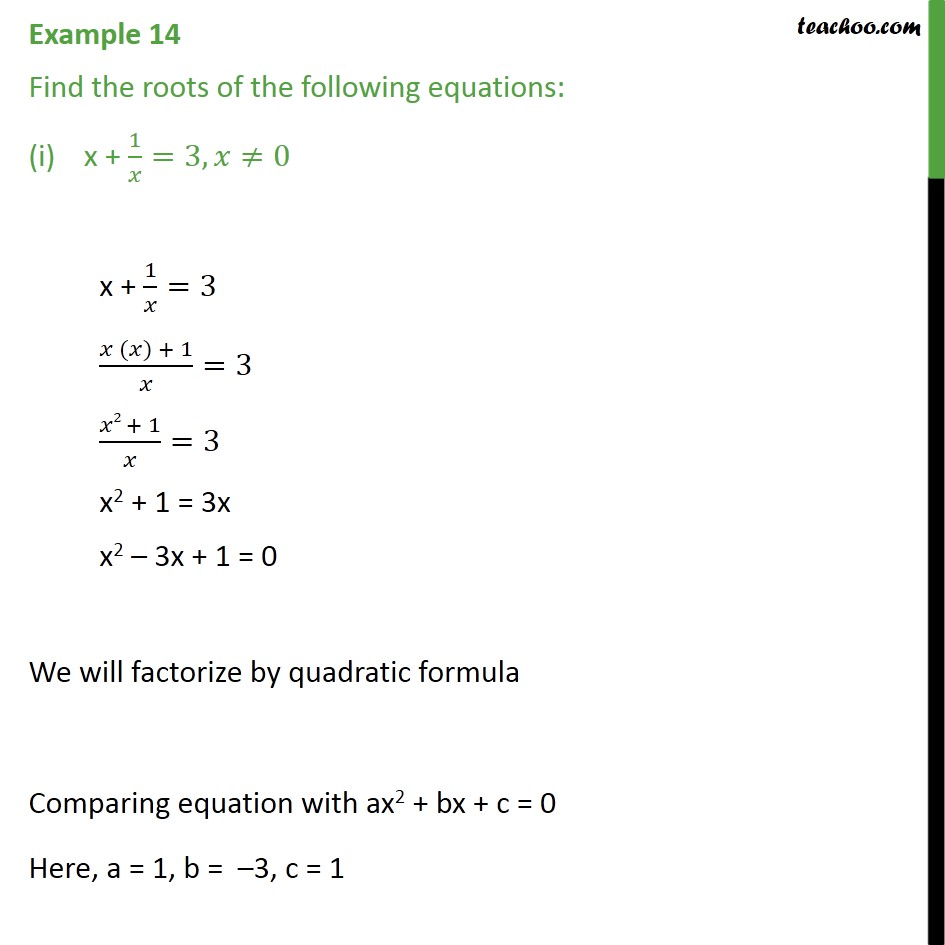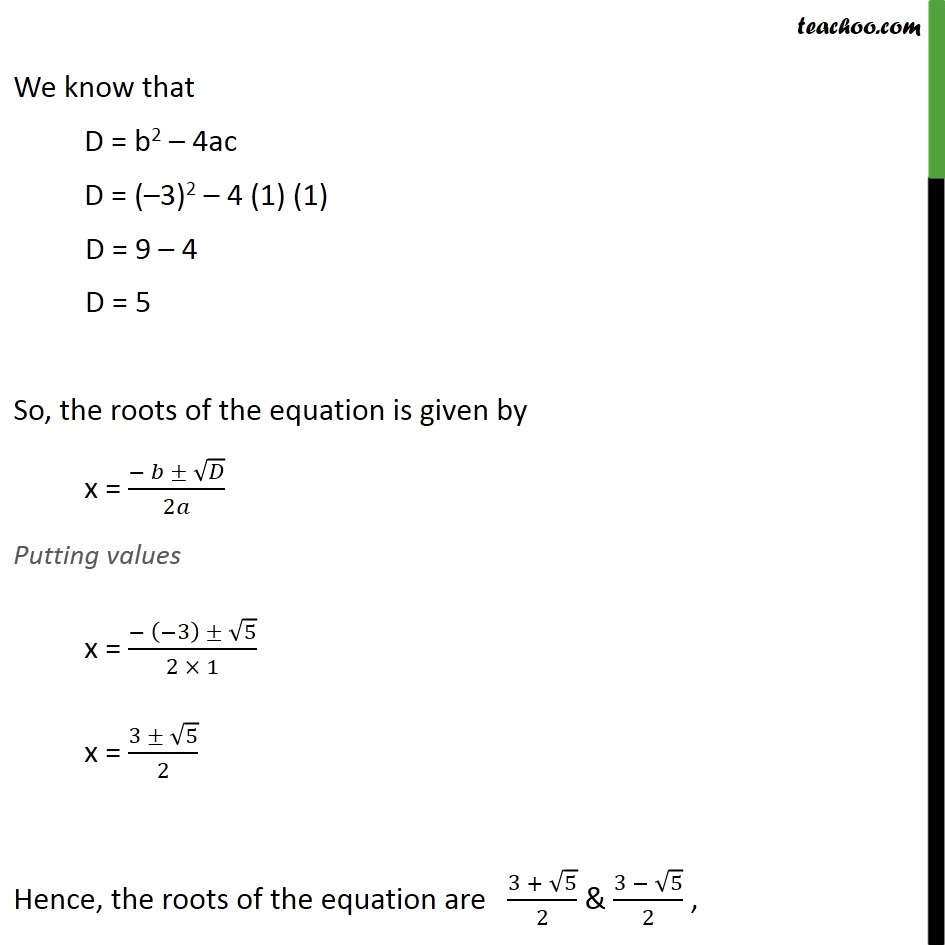1. Chapter 4 Class 10 Quadratic Equations (Term 2)
2. Concept wise
3. Solving by quadratic formula - Equation given

Transcript

Example 14 Find the roots of the following equations: (i) x + 1/𝑥=3,𝑥≠0 x + 1/𝑥=3 (𝑥 (𝑥) + 1)/𝑥=3 (𝑥2 + 1)/𝑥=3 x2 + 1 = 3x x2 – 3x + 1 = 0 We will factorize by quadratic formula Comparing equation with ax2 + bx + c = 0 Here, a = 1, b = –3, c = 1 We know that D = b2 – 4ac D = (–3)2 – 4 (1) (1) D = 9 – 4 D = 5 So, the roots of the equation is given by x = (− 𝑏 ± √𝐷)/2𝑎 Putting values x = (− (−3) ± √5)/(2 × 1) x = (3 ± √5)/2 Hence, the roots of the equation are (3 + √5)/2 & (3 − √5)/2 ,